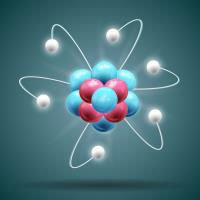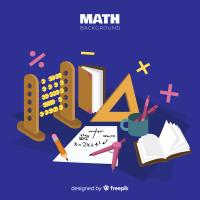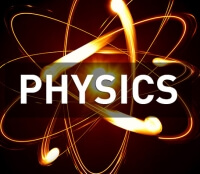Courses

# Chapter 21 : Speed of Light - HC Verma Solution, Physics Class 11 Notes | EduRev

## JEE: Chapter 21 : Speed of Light - HC Verma Solution, Physics Class 11 Notes | EduRev

The document Chapter 21 : Speed of Light - HC Verma Solution, Physics Class 11 Notes | EduRev is a part of the JEE Course Physics Class 11.
All you need of JEE at this link: JEE
``` Page 1

21.1
SOLUTIONS TO CONCEPTS
CHAPTER 21
1. In the given Fizeau’ ? apparatus,
D = 12 km = 12 × 10
3
m
n = 180
c = 3 × 10
8
m/sec
We know, c =
?
? Dn 2
? ? =
Dn 2
c ?
?
?
? 180
Dn 2
c
deg/sec
? ? =
180 10 24
10 3 180
3
8
? ?
? ?
= 1.25 × 10
4
deg/sec ?
2. In the given Focault experiment,
R = Distance between fixed and rotating mirror = 16m
? = Angular speed = 356 rev/ ? = 356 × 2 ? rad/sec
b = Distance between lens and rotating mirror = 6m
a = Distance between source and lens = 2m
s = shift in image = 0.7 cm = 0.7 × 10
–3
m
So, speed of light is given by,
C =
) b R ( s
a R 4
2
?
?
=
) 6 16 ( 10 7 . 0
2 2 356 16 4
3
2
? ?
? ? ? ? ?
?
= 2.975 × 10
8
m/s ?
3. In the given Michelson experiment,
D = 4.8 km = 4.8 × 10
3
m
N = 8
We know, c =
?
?
2
N D
? ? =
DN
c 2 ?
DN
c
rev/sec =
8 10 8 . 4
10 3
3
8
? ?
?
= 7.8 × 10
3
rev/sec
* * * * *
```Use Code STAYHOME200 and get INR 200 additional OFF Use Coupon Code
All Tests, Videos & Notes of JEE: JEE

### Top Courses for JEE## Physics Class 11

73 videos|330 docs|177 tests

### Top Courses for JEETrack your progress, build streaks, highlight & save important lessons and more!

,

,

,

,

,

,

,

,

,

,

,

,

,

,

,

,

,

,

,

,

,

,

,

,

;